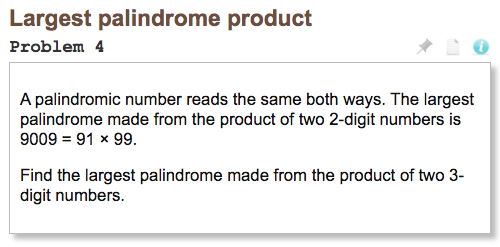# Project Euler Problem 4: Largest palindrome productProject Euler problem 4 feels like a step back in difficulty. The numbers involved aren’t too big so we don’t have to worry about resource constraints. The subproblems it breaks down into are fairly straightforward. If you haven’t yet, take some time with this problem on your own and continue on down below.

## Breaking the Problem Down

We can break this problem down into several subproblems:

1. Be able to check whether a number is a “palindrome”
2. Look at the right set of numbers and check whether each is a palindrome
3. Choose the right one from the final set

## Subproblem 1: The is_palindrome(n) Function

Let’s start with the first subproblem and write an is_palindrome(n) function to check whether a number is a palindrome. This function uses arithmetic to create a new integer with the digits of n reversed

def is_palindrome(n):
backwards = 0
temp = n
while temp > 0:
backwards *= 10
backwards += temp % 10
temp = temp // 10
return backwards == n


There are several ways you could define this is_palindrome(n) function. You might prefer to use strings rather than arithmetic:

def is_palindrome(n):
return str(n) == str(n)[::-1]


This one uses Python’s extended slicing, one of my favorite Python features. Slicing makes Python a joy to work with.

## Subproblem 2: Generating Products

How can we generate the set of numbers we need to check? We’re interested in all the products of two 3-digit numbers. We can use a simple for loop to generate each 3-digit number. To generate all pairs of 3-digit numbers we can nest two for loops:

products = []
for x in range(100, 1000):
for y in range(100, 1000):
products.append(x * y)


The nested for loops above will generate every permutation (specifically, the “permutation with repetition” or Cartesian product) of three digit numbers, use those factors to calculate the product, and add that product to a list of all products.

Python has a wonderful standard library. The itertools module ( docs) is particularly useful for Project Euler problems with how often it throws combinatorics problems at us.Here is the same solution using itertools.product and a list comprehension:

from itertools import product
factor_pairs = product(range(100, 1000), repeat=2)
products = [factors * factors for factors in factor_pairs]


### Speeding up with Combinations

The approach above using products will make us duplicate some of our work. For example, $101 * 202$ is the same as $202 * 101$, and the approach above calculates that product ($20{,}402$) multiple times. Wasted compute cycles, right?

It doesn’t matter much for this problem because the numbers are small enough to just power through, but it’s easy to imagine a situation where unnecessarily repeating work does cause problems.

Because the order of factors doesn’t matter, we can use a combination rather than a product to find all the products we want. We’ll skip straight to the itertools solution this time:

from itertools import combinations_with_replacement
factor_pairs = combinations_with_replacement(range(100, 1000), r=2)
products = [factors * factors for factors in factor_pairs]


This approach cuts the length of products about in half because it isn’t unnecessarily repeating calculations. That’s not critical for this problem, but being aware of these issues will help us down the road.

## Subproblem 3: Finding the Correct Product

Now that we’ve generated every product we want and stored them in a products list and have a is_palindrome(n) function we can filter down to just palindromic numbers:

palindromes = [product for product in products if is_palindrome(product)]


From there we can sort and take the largest palindrome:

print(sorted(palindromes)[-1])


This approach uses Python’s built-in sorted() function ( docs) and looks at the end of the list for the biggest value.

## Can you Improve it?

The solution described here generates all products of 3-digit numbers, even the small ones we know probably aren’t the answer. The nested for loops above count up through all the factors to generate products and then palindromes. It’s possible to write a solution that counts down and finds the answer with much less compute than the solution in this post. Can you figure out how?

See an issue on this page? Report a typo, bug, or give general feedback on GitHub.